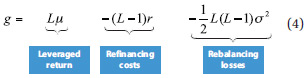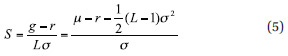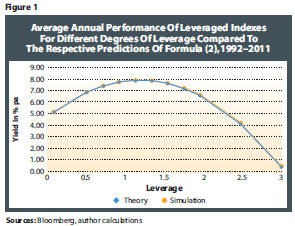Optimal Design Of Risk Control Strategy Indexes

August 17, 2012

However, as we show in the mathematical derivation in Appendix 2 online (the same result has been shown in Despande 2009 and Giese 2010), in the long run, the performance of a leveraged index is governed by the law of great numbers, and therefore the long-run return g of a leveraged index is very accurately described by the following simple relationship:In essence, the model equation (4) says that in the long run, the return of the leveraged index strategy is L times the return of the underlying equity index minus refinancing costs minus a term representing the adverse effect of rebalancing the portfolio on a continuous basis. In essence, this rebalancing loss comes from the well-known fact that the performance of leveraged indexes is path dependent.

This is a very important result, as it shows that leveraged ETFs on different underlying indexes and different leverage factors all exhibit the same long-run performance characteristics, which is essentially determined by three parameters:

1. The higher the yield ? of the underlying, the higher the more attractive leverage becomes.
2. The higher the average refinancing rate r, the higher the less attractive leverage is.
3. The higher the average realized volatility ? of the underlying, the higher the less attractive leverage becomes.

In other words, the complex path dependency of leveraged ETFs that is often cited as a main disadvantage of leveraged ETFs is absorbed in the long run into a simple rebalancing term that only depends on the average realized volatility of the underlying index during the investment period and the leverage factor of the index.

Based on the performance of leveraged indexes (4), we conclude the Sharpe ratio of a leveraged index isThe key observation from the Sharpe ratio (5) is that for a leveraged strategy (i.e., L>1), the Sharpe ratio turns out to be always smaller than the Sharpe ratio of the underlying index (2). The reasons for the deterioration in the Sharpe ratio are the aforementioned rebalancing losses, which reduce the outperformance of the leveraged index over the risk-free rate. Hence, even in strong bull markets where a leveraged index strongly outperforms a nonleveraged index in absolute terms, it will never outperform the nonleveraged index from a risk-adjusted perspective.

So far we have summarized theoretical results regarding leveraged indexes, which were derived in the online appendix based on stochastic calculus. This mathematical analysis is based on the model of equity returns following a normal distribution, in contrast to real-world equity returns, which are typically heavy tailed. Therefore, it is essential to verify the theoretical results by comparing them to real-world results. As a test case, we use the Euro Stoxx 50 Index and the EONIA money market rate as portfolio components.

Figure 1 compares the long-run returns of leveraged indexes for different degrees of leverage and compares them with the theoretical results obtained by the simple formula (4). The key observation is that the theoretical formula (2) provides a very accurate prediction of the long-run returns of leveraged indexes.In essence, the real-world backtests verify the fact that in the long run, the performance of leveraged indexes can be well understood according to the simple performance model (2) with a Sharpe ratio arrived at using formula (5).

To conclude, we have obtained the first key result of our analysis: Leveraged indexes without an embedded risk-control method (i.e., that do not respond to volatility in determining the size of the equity investment) have a Sharpe ratio that is always lower than the Sharpe ratio of the underlying equity index in the long run.